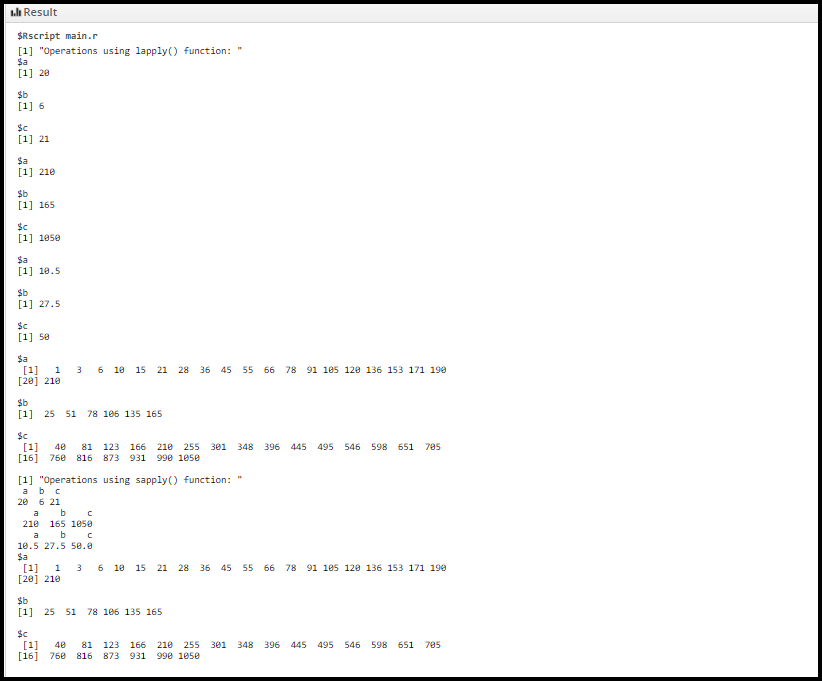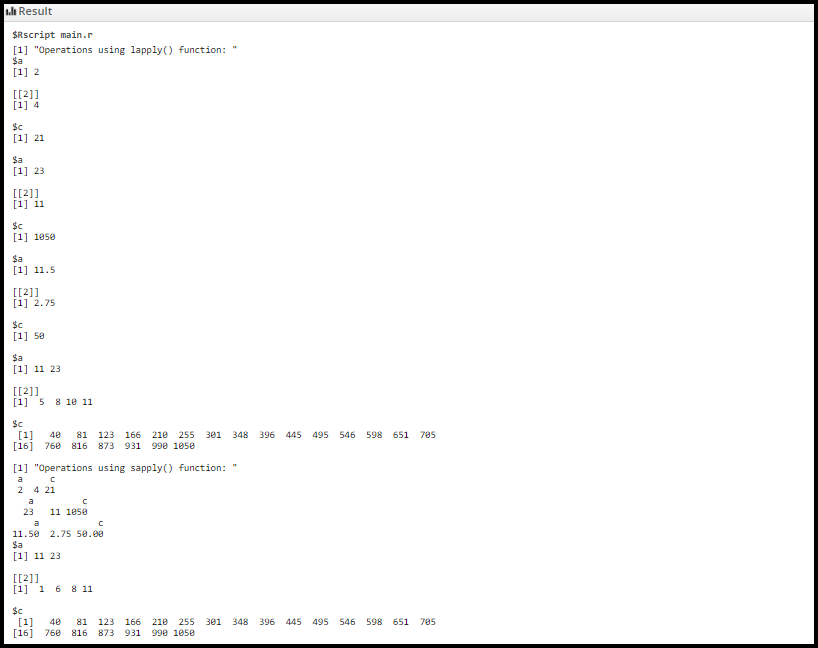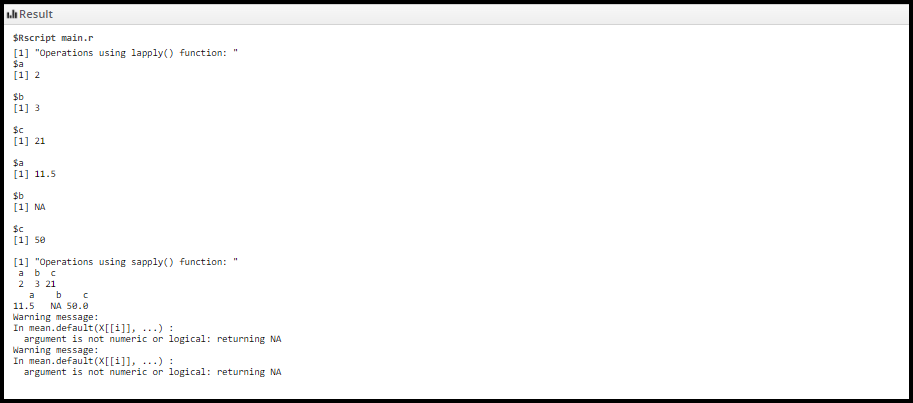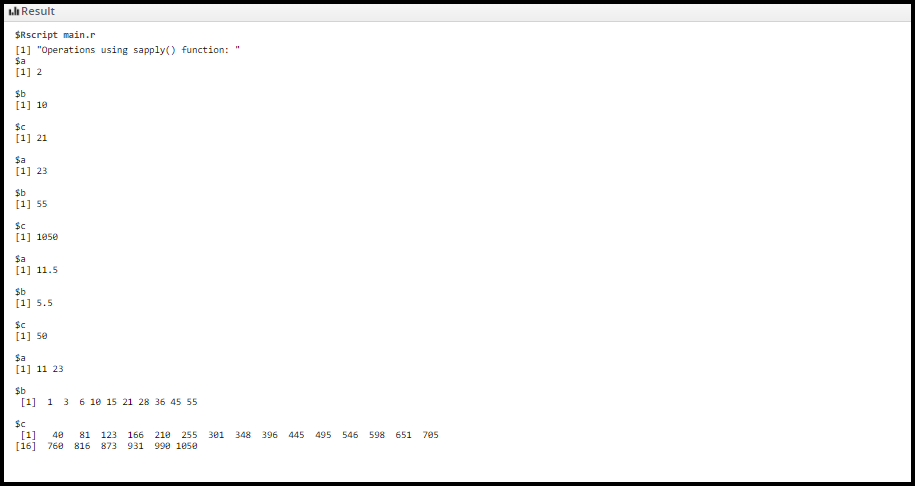Difference Between lapply() VS sapply() in R

• Difficulty Level : Hard
• Last Updated : 16 Jan, 2022

A list in R Programming Language can be passed as an argument in lapply() and sapply() functions. It is quite helpful to perform some of the general operations like calculation of sum, cumulative sum, mean, etc of the elements in the objects held by a list.

lapply()

Using “for” loop in R for iterating over a list or vector takes a lot of memory and it is quite slow also. And when it comes to dealing with large data set and iterating over them, for loop is not advised. R provides many alternatives to be applied to lists for looping operations that are pretty useful when working interactively on a command line. lapply() function is one of those functions and it is used to apply a function over a list.

Syntax: lapply(List, Operation)

Arguments:

• List: list containing a number of objects
• Operation: length, sum, mean, and cumsum

Return value: Returns a numeric value

lapply() function is used with a list and performs the following operations:

• lapply(List, length): Returns the length of objects present in the list, List.
• lapply(List, sum): Returns the sum of elements held by objects in the list, List.
• lapply(List, mean): Returns the mean of elements held by objects in the list, List.
• lapply(List, cumsum): Returns the cumulative sum of elements held by objects present inside the list, List.

sapply()

This function is also used to apply a function over a list but with simplified results.

Syntax: sapply(List, Operation)

Arguments:

• List: list containing a number of objects
• Operation: length, sum, mean, and cumsum

Return value: Returns a numeric value

lapply() function is used with a list and performs operations like:

• sapply(List, length): Returns the length of objects present in the list, List.
• sapply(List, sum): Returns the sum of elements held by objects in the list, List.
• sapply(List, mean): Returns the mean of elements held by objects in the list, List.
• sapply(List, cumsum): Returns the cumulative sum of elements held by objects present inside the list, List.

Difference between  lapply() and sapply() functions:

lapply() function displays the output as a list whereas sapply() function displays the output as a vector. lapply() and sapply() functions are used to perform some operations in a list of objects. sapply() function in R is more efficient than lapply() in the output returned because sapply() stores values directly into a vector.

Example 1:  The lapply() function returns the output as a list whereas sapply() function returns the output as a vector

R

 print("Operations using lapply() function: ") # Initializing list1# list1 have three objects a, b, and c# and they all are numeric objects (same data type)list1 <- list(a = 1: 20, b = 25:30, c = 40:60) # Printing the length of list1 objectslapply(list1, length) # Printing the sum of elements present in the# list1 objectslapply(list1, sum) # Printing the mean of elements present in the# list1 objectslapply(list1, mean) # Printing the cumulative sum of elements# present in the list1 objectslapply(list1, cumsum) print("Operations using sapply() function: ")  # Initializing list2# list2 have three objects a, b, and c# and they all are numeric objects (same data# type)list2 <- list(a = 1: 20, b = 25:30, c = 40:60) # Printing the length of list2 objectssapply(list2, length) # Printing the sum of elements# present in the list2 objectssapply(list2, sum) # Printing the mean of elements# present in the list2 objectssapply(list2, mean) # Printing the cumulative sum# of elements present in the list2 objectssapply(list2, cumsum)

Output:Example 2: As you can see in the output, The lapply() function returns the output as a list whereas sapply() function returns the output as a vector.

R

 print("Operations using lapply() function: ")  # Initializing list1# list1 have three objects a, b, and c# and they all are numeric objects (same data type)list1 <- list(a=11: 12, sample(c(1, 2, 5, 3),                               size=4, replace=FALSE),              c=40: 60) # Printing the length of list1 objectslapply(list1, length) # Printing the sum of elements present in the# list1 objectslapply(list1, sum) # Printing the mean of elements present in the# list1 objectslapply(list1, mean) # Printing the cumulative sum of elements present# in the list1 objectslapply(list1, cumsum) print("Operations using sapply() function: ") # Initializing list2# list2 have three objects a, b, and c# and they all are numeric objects (same data type)list2 <- list(a=11: 12, sample(c(1, 2, 5, 3),                               size=4, replace=FALSE),              c=40: 60) # Printing the length of list2 objectssapply(list2, length) # Printing the sum of elements# present in the list2 objectssapply(list2, sum) # Printing the mean of elements# present in the list2 objectssapply(list2, mean) # Printing the cumulative sum of# elements present in the list2 objectssapply(list2, cumsum)

Output:Example 3: We can use non-numeric objects also in a list but after applying operations like “mean” on the list we get the “NA” result in output since these operations work for numeric objects only.

R

 print("Operations using lapply() function: ") # Initializing list1# list1 have three objects a, b, and c# and they all are numeric objects# (same data type)list1 <- list(a = 11: 12, b = c('Geeks', 'for', 'Geeks'),              c = 40:60) # Printing the length of list1 objectslapply(list1, length) # Printing the mean of elements# present in the list1 objectslapply(list1, mean) print("Operations using sapply() function: ") # Initializing list2# list2 have three objects a, b, and c# and they all are numeric objects (same data type)list2 <- list(a = 11: 12, b = c('Geeks', 'for', 'Geeks'),              c = 40:60) # Printing the length of list2 objectssapply(list2, length) # Printing the mean of elements# present in the list2 objectssapply(list2, mean)

Output:The lapply() and sapply() functions print NA for object b in list1 since b is a non-numeric object. R compiler gives a warning whenever we apply these operations on a list containing a number of non-numeric objects.

Returning output as a list using sapply() function

sapply() function accepts a third argument using which we can return the output as a list instead of a vector.

Syntax: sapply(List, Operation, simplify = FALSE)

Arguments:

• List: list containing a number of objects
• Operation: length, sum, mean, and cumsum
• simplify = FALSE: Returns the output as a list instead of a vector

Return value: Returns a numeric value.

R

 print("Operations using sapply() function: ") # list1 have three objects a, b, and c# and they all are numeric objects (same data type)list1 <- list(a = 11:12, b = 1:10, c = 40:60) # Printing the length of list1 objects as a listsapply(list1, length, simplify = FALSE) # Printing the sum of elements present# in the list1 objects as a listsapply(list1, sum, simplify = FALSE) # Printing the mean of elements present# in the list1 objects as a listsapply(list1, mean, simplify = FALSE) # Printing the cumulative sum of elements# present in the list1 objects as a listsapply(list1, cumsum, simplify = FALSE)

Output:As we can see in the output, sapply() function returns the output as a list instead of a vector. So, we can always the third argument to the sapply() function whenever we need to print the output as a list instead of a vector.

My Personal Notes arrow_drop_up